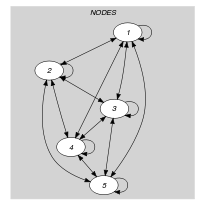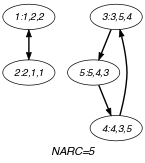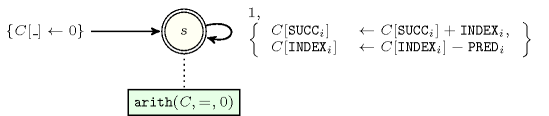## 5.199. inverse

Origin

CHIP

Constraint

$\mathrm{𝚒𝚗𝚟𝚎𝚛𝚜𝚎}\left(\mathrm{𝙽𝙾𝙳𝙴𝚂}\right)$

Synonyms

$\mathrm{𝚊𝚜𝚜𝚒𝚐𝚗𝚖𝚎𝚗𝚝}$, $\mathrm{𝚌𝚑𝚊𝚗𝚗𝚎𝚕}$, $\mathrm{𝚒𝚗𝚟𝚎𝚛𝚜𝚎}_\mathrm{𝚌𝚑𝚊𝚗𝚗𝚎𝚕𝚒𝚗𝚐}$.

Argument
 $\mathrm{𝙽𝙾𝙳𝙴𝚂}$ $\mathrm{𝚌𝚘𝚕𝚕𝚎𝚌𝚝𝚒𝚘𝚗}\left(\mathrm{𝚒𝚗𝚍𝚎𝚡}-\mathrm{𝚒𝚗𝚝},\mathrm{𝚜𝚞𝚌𝚌}-\mathrm{𝚍𝚟𝚊𝚛},\mathrm{𝚙𝚛𝚎𝚍}-\mathrm{𝚍𝚟𝚊𝚛}\right)$
Restrictions
 $\mathrm{𝚛𝚎𝚚𝚞𝚒𝚛𝚎𝚍}$$\left(\mathrm{𝙽𝙾𝙳𝙴𝚂},\left[\mathrm{𝚒𝚗𝚍𝚎𝚡},\mathrm{𝚜𝚞𝚌𝚌},\mathrm{𝚙𝚛𝚎𝚍}\right]\right)$ $\mathrm{𝙽𝙾𝙳𝙴𝚂}.\mathrm{𝚒𝚗𝚍𝚎𝚡}\ge 1$ $\mathrm{𝙽𝙾𝙳𝙴𝚂}.\mathrm{𝚒𝚗𝚍𝚎𝚡}\le |\mathrm{𝙽𝙾𝙳𝙴𝚂}|$ $\mathrm{𝚍𝚒𝚜𝚝𝚒𝚗𝚌𝚝}$$\left(\mathrm{𝙽𝙾𝙳𝙴𝚂},\mathrm{𝚒𝚗𝚍𝚎𝚡}\right)$ $\mathrm{𝙽𝙾𝙳𝙴𝚂}.\mathrm{𝚜𝚞𝚌𝚌}\ge 1$ $\mathrm{𝙽𝙾𝙳𝙴𝚂}.\mathrm{𝚜𝚞𝚌𝚌}\le |\mathrm{𝙽𝙾𝙳𝙴𝚂}|$ $\mathrm{𝙽𝙾𝙳𝙴𝚂}.\mathrm{𝚙𝚛𝚎𝚍}\ge 1$ $\mathrm{𝙽𝙾𝙳𝙴𝚂}.\mathrm{𝚙𝚛𝚎𝚍}\le |\mathrm{𝙽𝙾𝙳𝙴𝚂}|$
Purpose

Enforce each vertex of a digraph to have exactly one predecessor and one successor. In addition the following two statements are equivalent:

1. The successor of the ${i}^{th}$ node is the ${j}^{th}$ node.

2. The predecessor of the ${j}^{th}$ node is the ${i}^{th}$ node.

Example
$\left(\begin{array}{c}〈\begin{array}{ccc}\mathrm{𝚒𝚗𝚍𝚎𝚡}-1\hfill & \mathrm{𝚜𝚞𝚌𝚌}-2\hfill & \mathrm{𝚙𝚛𝚎𝚍}-2,\hfill \\ \mathrm{𝚒𝚗𝚍𝚎𝚡}-2\hfill & \mathrm{𝚜𝚞𝚌𝚌}-1\hfill & \mathrm{𝚙𝚛𝚎𝚍}-1,\hfill \\ \mathrm{𝚒𝚗𝚍𝚎𝚡}-3\hfill & \mathrm{𝚜𝚞𝚌𝚌}-5\hfill & \mathrm{𝚙𝚛𝚎𝚍}-4,\hfill \\ \mathrm{𝚒𝚗𝚍𝚎𝚡}-4\hfill & \mathrm{𝚜𝚞𝚌𝚌}-3\hfill & \mathrm{𝚙𝚛𝚎𝚍}-5,\hfill \\ \mathrm{𝚒𝚗𝚍𝚎𝚡}-5\hfill & \mathrm{𝚜𝚞𝚌𝚌}-4\hfill & \mathrm{𝚙𝚛𝚎𝚍}-3\hfill \end{array}〉\hfill \end{array}\right)$

The $\mathrm{𝚒𝚗𝚟𝚎𝚛𝚜𝚎}$ constraint holds since:

• $\mathrm{𝙽𝙾𝙳𝙴𝚂}\left[1\right].\mathrm{𝚜𝚞𝚌𝚌}=2⇔\mathrm{𝙽𝙾𝙳𝙴𝚂}\left[2\right].\mathrm{𝚙𝚛𝚎𝚍}=1$,

• $\mathrm{𝙽𝙾𝙳𝙴𝚂}\left[2\right].\mathrm{𝚜𝚞𝚌𝚌}=1⇔\mathrm{𝙽𝙾𝙳𝙴𝚂}\left[1\right].\mathrm{𝚙𝚛𝚎𝚍}=2$,

• $\mathrm{𝙽𝙾𝙳𝙴𝚂}\left[3\right].\mathrm{𝚜𝚞𝚌𝚌}=5⇔\mathrm{𝙽𝙾𝙳𝙴𝚂}\left[5\right].\mathrm{𝚙𝚛𝚎𝚍}=3$,

• $\mathrm{𝙽𝙾𝙳𝙴𝚂}\left[4\right].\mathrm{𝚜𝚞𝚌𝚌}=3⇔\mathrm{𝙽𝙾𝙳𝙴𝚂}\left[3\right].\mathrm{𝚙𝚛𝚎𝚍}=4$,

• $\mathrm{𝙽𝙾𝙳𝙴𝚂}\left[5\right].\mathrm{𝚜𝚞𝚌𝚌}=4⇔\mathrm{𝙽𝙾𝙳𝙴𝚂}\left[4\right].\mathrm{𝚙𝚛𝚎𝚍}=5$.

Typical
$|\mathrm{𝙽𝙾𝙳𝙴𝚂}|>1$
Symmetries
• Items of $\mathrm{𝙽𝙾𝙳𝙴𝚂}$ are permutable.

• Attributes of $\mathrm{𝙽𝙾𝙳𝙴𝚂}$ are permutable w.r.t. permutation $\left(\mathrm{𝚒𝚗𝚍𝚎𝚡}\right)$ $\left(\mathrm{𝚜𝚞𝚌𝚌},\mathrm{𝚙𝚛𝚎𝚍}\right)$ (permutation applied to all items).

Arg. properties
• Functional dependency: $\mathrm{𝙽𝙾𝙳𝙴𝚂}.\mathrm{𝚜𝚞𝚌𝚌}$ determined by $\mathrm{𝙽𝙾𝙳𝙴𝚂}.\mathrm{𝚒𝚗𝚍𝚎𝚡}$ and $\mathrm{𝙽𝙾𝙳𝙴𝚂}.\mathrm{𝚙𝚛𝚎𝚍}$.

• Functional dependency: $\mathrm{𝙽𝙾𝙳𝙴𝚂}.\mathrm{𝚙𝚛𝚎𝚍}$ determined by $\mathrm{𝙽𝙾𝙳𝙴𝚂}.\mathrm{𝚒𝚗𝚍𝚎𝚡}$ and $\mathrm{𝙽𝙾𝙳𝙴𝚂}.\mathrm{𝚜𝚞𝚌𝚌}$.

Usage

This constraint is used in order to make the link between the successor and the predecessor variables. This is sometimes required by specific heuristics that use both predecessor and successor variables. In some problems, the $\mathrm{successor}$ and $\mathrm{predecessor}$ variables are respectively interpreted as column an row variables (i.e., we have a bijection between the $\mathrm{successor}$ variables and their values). This is for instance the case in the $n$-queens problem (i.e., place $n$ queens on an $n$ by $n$ chessboard in such a way that no two queens are on the same row, the same column or the same diagonal) when we use the following model: to each column of the chessboard we associate a variable that gives the row where the corresponding queen is located. Symmetrically, to each row of the chessboard we create a variable that indicates the column where the associated queen is placed. Having these two sets of variables, we can now write a heuristics that selects the column or the row for which we have the fewest number of alternatives for placing a queen.

Remark

In the original $\mathrm{𝚒𝚗𝚟𝚎𝚛𝚜𝚎}$ constraint of CHIP the $\mathrm{𝚒𝚗𝚍𝚎𝚡}$ attribute was not explicitly present. It was implicitly defined as the position of a variable in a list, the first position being 1. This is also the case for SICStus Prolog, JaCoP and Gecode where the variables are respectively indexed from 1, 0 and 0. Within SICStus Prolog and JaCoP (http://www.jacop.eu/), the $\mathrm{𝚒𝚗𝚟𝚎𝚛𝚜𝚎}$ constraint is called $\mathrm{𝚊𝚜𝚜𝚒𝚐𝚗𝚖𝚎𝚗𝚝}$. Within Gecode, it is called $\mathrm{𝚌𝚑𝚊𝚗𝚗𝚎𝚕}$ (http://www.gecode.org/).

Algorithm

An arc-consistency filtering algorithm for the $\mathrm{𝚒𝚗𝚟𝚎𝚛𝚜𝚎}$ constraint is described in [Cymer12], [CymerPhD13]. The algorithm is based on the following ideas:

• We first normalize the domains of the variables by removing value $i$ from the ${j}^{th}$ $\mathrm{predecessor}$ variable if value $j$ does not belong to the ${i}^{th}$ $\mathrm{successor}$ variable, and by removing value $j$ from the ${i}^{th}$ $\mathrm{successor}$ variable if value $i$ does not belong to the ${j}^{th}$ $\mathrm{predecessor}$ variable.

• Second, one can map solutions to the $\mathrm{𝚒𝚗𝚟𝚎𝚛𝚜𝚎}$ constraint to perfect matchings in a so-called variable bipartite graph derived from the domain of the variables of the constraint in the following way: to each successor variable corresponds a vertex; similarly to each predecessor variable corresponds a vertex; there is and edge between the ${i}^{th}$ $\mathrm{successor}$ variable and the ${j}^{th}$ $\mathrm{predecessor}$ variable if and only if value $i$ belongs to the domain of the ${j}^{th}$ $\mathrm{predecessor}$ variable and value $j$ belongs to the domain of the ${i}^{th}$ $\mathrm{successor}$ variable.

• Third, Dulmage-Mendelsohn decomposition [DulmageMendelsohn58] is used to characterise all edges that do not belong to any perfect matching, and therefore prune the corresponding variables.

Systems

generalisation: $\mathrm{𝚒𝚗𝚟𝚎𝚛𝚜𝚎}_\mathrm{𝚘𝚏𝚏𝚜𝚎𝚝}$ (do not assume anymore that the smallest value of the $\mathrm{𝚙𝚛𝚎𝚍}$ or $\mathrm{𝚜𝚞𝚌𝚌}$ attributes is equal to 1), $\mathrm{𝚒𝚗𝚟𝚎𝚛𝚜𝚎}_\mathrm{𝚜𝚎𝚝}$ ($\mathrm{𝚍𝚘𝚖𝚊𝚒𝚗}\mathrm{𝚟𝚊𝚛𝚒𝚊𝚋𝚕𝚎}$ replaced by $\mathrm{𝚜𝚎𝚝}$ $\mathrm{𝚟𝚊𝚛𝚒𝚊𝚋𝚕𝚎}$), $\mathrm{𝚒𝚗𝚟𝚎𝚛𝚜𝚎}_\mathrm{𝚠𝚒𝚝𝚑𝚒𝚗}_\mathrm{𝚛𝚊𝚗𝚐𝚎}$ (partial mapping between two collections of distinct size).

Keywords
Arc input(s)

$\mathrm{𝙽𝙾𝙳𝙴𝚂}$

Arc generator
$\mathrm{𝐶𝐿𝐼𝑄𝑈𝐸}$$↦\mathrm{𝚌𝚘𝚕𝚕𝚎𝚌𝚝𝚒𝚘𝚗}\left(\mathrm{𝚗𝚘𝚍𝚎𝚜}\mathtt{1},\mathrm{𝚗𝚘𝚍𝚎𝚜}\mathtt{2}\right)$

Arc arity
Arc constraint(s)
 $•\mathrm{𝚗𝚘𝚍𝚎𝚜}\mathtt{1}.\mathrm{𝚜𝚞𝚌𝚌}=\mathrm{𝚗𝚘𝚍𝚎𝚜}\mathtt{2}.\mathrm{𝚒𝚗𝚍𝚎𝚡}$ $•\mathrm{𝚗𝚘𝚍𝚎𝚜}\mathtt{2}.\mathrm{𝚙𝚛𝚎𝚍}=\mathrm{𝚗𝚘𝚍𝚎𝚜}\mathtt{1}.\mathrm{𝚒𝚗𝚍𝚎𝚡}$
Graph property(ies)
$\mathrm{𝐍𝐀𝐑𝐂}$$=|\mathrm{𝙽𝙾𝙳𝙴𝚂}|$

Graph model

In order to express the binary constraint that links two vertices one has to make explicit the identifier of the vertices. This is why the $\mathrm{𝚒𝚗𝚟𝚎𝚛𝚜𝚎}$ constraint considers objects that have three attributes:

• One fixed attribute $\mathrm{𝚒𝚗𝚍𝚎𝚡}$ that is the identifier of the vertex,

• One variable attribute $\mathrm{𝚜𝚞𝚌𝚌}$ that is the successor of the vertex,

• One variable attribute $\mathrm{𝚙𝚛𝚎𝚍}$ that is the predecessor of the vertex.

Parts (A) and (B) of Figure 5.199.1 respectively show the initial and final graph associated with the Example slot. Since we use the $\mathrm{𝐍𝐀𝐑𝐂}$ graph property, the arcs of the final graph are stressed in bold.

##### Figure 5.199.1. Initial and final graph of the $\mathrm{𝚒𝚗𝚟𝚎𝚛𝚜𝚎}$ constraint(a) (b)
Signature

Since all the $\mathrm{𝚒𝚗𝚍𝚎𝚡}$ attributes of the $\mathrm{𝙽𝙾𝙳𝙴𝚂}$ collection are distinct and because of the first condition $\mathrm{𝚗𝚘𝚍𝚎𝚜}\mathtt{1}.\mathrm{𝚜𝚞𝚌𝚌}=\mathrm{𝚗𝚘𝚍𝚎𝚜}\mathtt{2}.\mathrm{𝚒𝚗𝚍𝚎𝚡}$ of the arc constraint all the vertices of the final graph have at most one predecessor.

Since all the $\mathrm{𝚒𝚗𝚍𝚎𝚡}$ attributes of the $\mathrm{𝙽𝙾𝙳𝙴𝚂}$ collection are distinct and because of the second condition $\mathrm{𝚗𝚘𝚍𝚎𝚜}\mathtt{2}.\mathrm{𝚙𝚛𝚎𝚍}=\mathrm{𝚗𝚘𝚍𝚎𝚜}\mathtt{1}.\mathrm{𝚒𝚗𝚍𝚎𝚡}$ of the arc constraint all the vertices of the final graph have at most one successor.

From the two previous remarks it follows that the final graph is made up from disjoint paths and disjoint circuits. Therefore the maximum number of arcs of the final graph is equal to its maximum number of vertices $\mathrm{𝙽𝙾𝙳𝙴𝚂}$. So we can rewrite the graph property $\mathrm{𝐍𝐀𝐑𝐂}=|\mathrm{𝙽𝙾𝙳𝙴𝚂}|$ to $\mathrm{𝐍𝐀𝐑𝐂}\ge |\mathrm{𝙽𝙾𝙳𝙴𝚂}|$ and simplify $\underline{\overline{\mathrm{𝐍𝐀𝐑𝐂}}}$ to $\overline{\mathrm{𝐍𝐀𝐑𝐂}}$.

Automaton

Figure 5.199.2 depicts the automaton associated with the $\mathrm{𝚒𝚗𝚟𝚎𝚛𝚜𝚎}$ constraint. To each item of the collection $\mathrm{𝙽𝙾𝙳𝙴𝚂}$ corresponds a signature variable ${S}_{i}$ that is equal to 1.

##### Figure 5.199.2. Automaton of the $\mathrm{𝚒𝚗𝚟𝚎𝚛𝚜𝚎}$ constraint